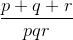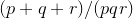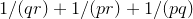Search IntMath
Close

450+ Math Lessons written by Math Professors and Teachers

5 Million+ Students Helped Each Year

1200+ Articles Written by Math Educators and Enthusiasts

Simplifying and Teaching Math for Over 23 Years

# IntMath Newsletter: squaring circle, lens fillet, data visualization

By Murray Bourne, 16 Feb 2016

16 Feb 2016

1. Squaring the circle - a reader's approach
2. IntMath Forum - latest post
3. Resource: The best Data Visualization tools
4. Math puzzles
5. Math movies: 8 videos that prove math is awesome
6. Final thought: Preparation for tomorrow

## 1. Squaring the circle - a reader's approachHere's a reader's approach to the age-old problem of constructing a square with the same area as a given circle, called "squaring the circle" Squaring the circle - a reader's approach

Here's a neat alternative approach in a related article from Jonathan Crabtree:

How to square a circle with string

Of course, the title of the IntMath blog, SquareCirclez, is named after this interesting issue.

## 2. IntMath Forum - latest post

Here's an interesting real-life application of conic sections and solving simultaneous equations.When manufacturing eyeglass lenses, it's sometimes necessary to produce a curved edge called a fillet (or "round"). A reader asked how to find the center and radius of such a fillet in the IntMath Forum.

## 3. Resource: Big Data Visualization - Review of the 20 Best Tools

A key 21st century math skill — and one which will certainly provide emplyment opportunities in the future — is the presentation and interpretation of data.There are many easy to use data visualization tools, including Tableau, Infogram, Plot.ly and Google Charts. Students should be learning how to use such tools, how to best present data meaningfully, and how to interpret visual data.

Here's a review of 20 such tools by Blueframe. Most of the tools have a free option and/or education pricing, and most are mobile friendly.

Big Data Visualization: Review of the 20 Best Tools

## 4. Math puzzles

The puzzle in the last IntMath Newsletter asked about the change in area when we decrease and increase side lengths of a rectangle.

Correct answers with sufficient explanation were given by: Francis, Rutwik, Kai, Jessica, Tomas, Andrzej, Nicos, Michael, Guido, Nour and Janet.

### New math puzzle

If p, q, r are distinct integers from 1 to 9 inclusively, what is the largest possible value of?

You can leave your responses here.

## 5. Math movies: 8 videos that prove math is awesomeI'm not sure that the word "prove" is especially appropriate here, but I think you'll find at least some of these worth a look. The collection is by Mashable: 8 Videos That Prove Math Is Awesome

## 6. Final thought: Preparation for tomorrow

American author H. Jackson Brown Jr wrote a book for his college-bound son in which he included 511 reminders about "how to live a happy and rewarding life".

The book went on to become a New York Times bestseller entitled Life's Little Instruction Book.

Here are 2 great quotes from that book:

"The best preparation for tomorrow is doing your best today."

"Live so that when your children think of fairness, caring, and integrity, they think of you."

That second quote could also have been addressed to teachers.

Until next time, enjoy whatever you learn.

### 20 Comments on “IntMath Newsletter: squaring circle, lens fillet, data visualization”

1. N MARAMBI says:

The largest value is 1. taking p+q+r = 1 + 2 +3 = 6 AND p * q * r = 6 . 1,2,and 3 gives the smallest denominator which results in the largest value.

2. Shihab says:

one (1)

3. Don Miller says:

ans =1
ratio p+q+r/pqr = 1/pq +1/pr + 1/qr For 1,2,3
= .5 + .333.. + .166..
= 1
Larger values of p,q,r yield smaller values. Therefor
1 is largest ratio and p,q,r have values 1,2,3

The maximum value of (p + q + r)/pqr is 1. This can be verified through:

1 + 2 + 3 = 6
1 X 2 X 3 = 6

Thus: 6/6 = 1

5. Ramesh babu says:

p=1,q=2 and r =3. Then the answer will be 1.
(1+2+3)/1*2*3 = 1.

6. Mayank Jain says:

The values would be 1, 2 and 3 (Since they have to be distinct...... max value is 1) for the values of P Q and R. This is because as the values of numbers increase the fraction as a whole decreases.

7. Nitin Deshpande says:

Let p+q+r = S, p.q.r = T, and L = S/T. The value of L can be maximum when the value of T is minimum.

The value of T is minimum when the value of p=1, q=2 and r=3.
The value of T=6, and value of S=6, hence the value of L=1, which is maximum.

8. george says:

(1+2+3)/(1*2*3)

9. Nour Hlwani says:

We have:

LaTeX: syntax error (Unable to compile LaTeX formula,
1<=p<=9
Check syntax.)

LaTeX: syntax error (Unable to compile LaTeX formula,
1<=q<=9
Check syntax.)

LaTeX: syntax error (Unable to compile LaTeX formula,
1<=r<=9
Check syntax.)

We need:=Where:

LaTeX: syntax error (Unable to compile LaTeX formula,
1<=qr<=9^2
Check syntax.)

And

LaTeX: syntax error (Unable to compile LaTeX formula,
1/(9^2)<=1/(qr)<=1
Check syntax.)

pr and pq are just the same we will have then:

LaTeX: syntax error (Unable to compile LaTeX formula,
1/27<=1/(qr) + 1/(pr) + 1/(pq)<=3
Check syntax.)

Same as:

LaTeX: syntax error (Unable to compile LaTeX formula,
1/27<=(p+q+r)/(pqr)<=3
Check syntax.)

The largest value would be 3 when p=q=r=1 .This value will decrease when p,q or r increase.

10. Tomas Garza says:

Suppose p < q < r. Then the numerator p + q + r is a linear function of p for any choice of p (1, 2,..., 7). The denominator p q r is a polynomial of degree 3 with positive coefficients and 0 constant term. Hence it is always greater that the numerator, i.e., the ratio is less than 1, except when p =1, q = 2 and r = 3, when the ratio is equal to 1. This means that the maximum is obtained when p = 1, q = 2, r = 3.

11. Christian Luca says:

The largest possible value of (p+q+r)/(p*q*r), with p, q, and r being distinct integers from 1 to 9 would be 1, for p=1, q=2, r=3, since any other combination of integers would yield a a numerator whose sum p+q+r is less than the denominator with product p*q*r. For the case p=1, q=2, r=3, however, the numerator and denominator are equal, with them both equal to 6.

12. Dineth says:

if p=q=r allows, max is when p,q,r = 1. (answer is 3)
whenever we add more than 1, and divide, it takes something out from the total. (as multiplication earns larger than sum of it for n>1)

if p=q=r not allows (DISTINCT)..
when p,q,r = [1,2,3] earns the max.

so six possible ways
p q r (p+q+r)/pqr
1 2 3 1.00
1 3 2 1.00
2 1 3 1.00
2 3 1 1.00
3 1 2 1.00
3 2 1 1.00

13. narender singh says:

the value of p,q,r is 1,2,3 which gives the maximum value

14. wesen says:

the largest possible value of (p+q+r)/(pqr) is one(1) when p=1 q=2,r=3

15. wesen says:

the largest possible value of p+q+r/pqr=1. when p=1, q=2, r=3.

16. Ben Murray says:

The largest total is 1, when P, Q & R are 1, 2 & 3 (in any order). As P, Q & R get bigger, the denominator (multiples) can only get bigger relative to the numerator (sums).

When P, Q & R are 1, 2 & 3, we see that the numerator and denominator are both 6; this is because 6 is a Perfect Number, a number that is equal to the sum of its proper positive divisors.

17. Γιώργος Βαρελάς says:

p > 1 or p = 1, q > 1 or q = 1, r > 1 or r = 1. pq > 1 or pq = 1, qr > 1 or qr = 1, rp > 1 or rp = 1. 1/(pq) < 1 or 1/(pq) = 1, 1/(qr) < 1 or 1/(qr) = 1, 1/(rp) < 1 or 1/(rp) = 1.
(p + q + r)/pqr =1/(qr) + 1/(rp) + 1/(pq) < 1 + 1 + 1 = 3, or [1/(qr) + 1/(rp) + 1/(pq)] = 1 + 1 + 1 = 3. [(p + q + r)/pqr]max = 3.
p = q = r = 1, because: [1/(qr)]max = [1/(rp)]max = [1/(pq)]max = 1, when: pmin = qmin = rmin = 1
If: p < q < r, then [(p + q + r)/pqr]max = 1, p = 1, q = 2, r = 3}:
pmin = 1, qmin = 2, rmin = 3. (pq)min = 2, (qr)min = 6, (rp)min = 3. [1/(pq)]max = 1/2, [1/(qr)]max = 1/6, [1/(rp)]max = 1/3.
(p + q + r)/pqr = [1/(qr) + 1/(rp) + 1/(pq)]max = 1/2 + 1/6 + 1/3 = 1. [(p + q + r)/pqr]max = 1.
p = 1, q = 2, r = 3, because: [1/(qr)]max = ½, [1/(rp)]max = 1/6, [1/(pq)]max = 1/3, when: pmin = 1, qmin = 2, rmin = 3.

18. Deepak says:

p=1, q=2, r=3

(p+q+r)/pqr = 6/6 = 1

Hence, if p, q, r are distinct integers from 1 to 9 inclusively, largest possible value of (p+q+r)/pqr is 1.

19. karimazmy says:

p=1q=2r=3

p+q+r=6

pqr=6
6/6=1

20. Darrrell says:

MY ANSWER FOR THIS MONTH'S PROBLEM

The largest value for the fraction is 1 ... This occurs when p, q, and r take on the 6 permutations of { 1, 2, 3 ) ... This problems shows how "multiplication" overpowers "addition" for positive integers ...

### Comment Preview

HTML: You can use simple tags like <b>, <a href="...">, etc.

To enter math, you can can either:

1. Use simple calculator-like input in the following format (surround your math in backticks, or qq on tablet or phone):
a^2 = sqrt(b^2 + c^2)
(See more on ASCIIMath syntax); or
2. Use simple LaTeX in the following format. Surround your math with $$ and $$.
$$\int g dx = \sqrt{\frac{a}{b}}$$
(This is standard simple LaTeX.)

NOTE: You can mix both types of math entry in your comment.